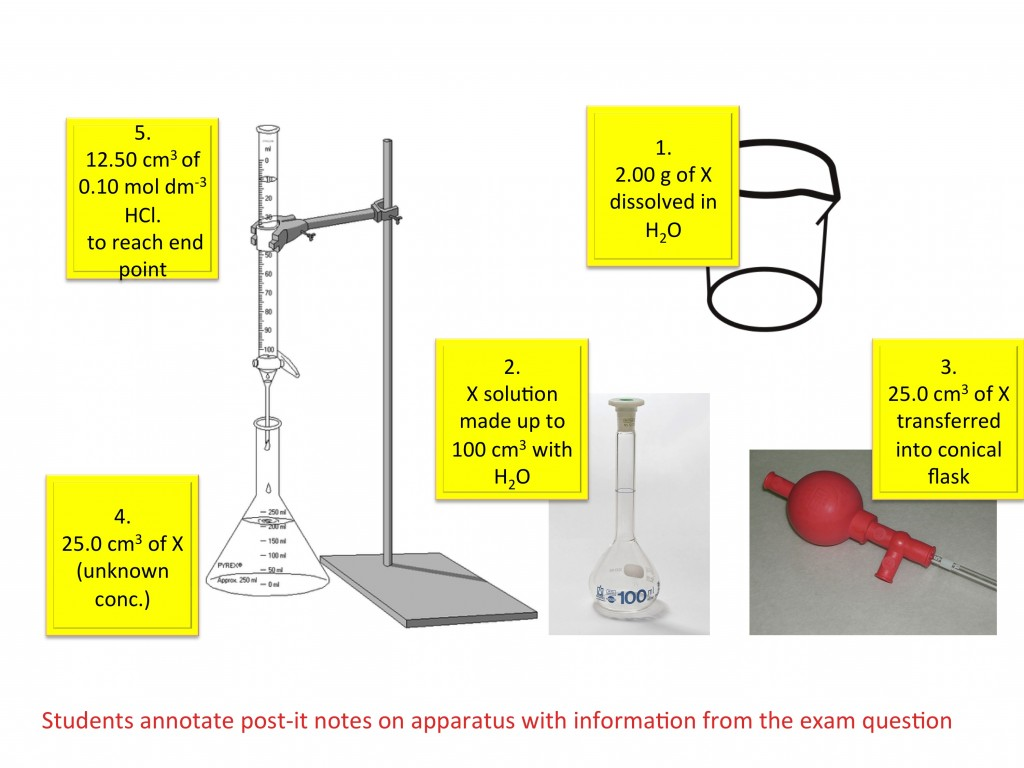# Concentration and titrations teaching resources

###### Worksheets and lesson ideas to challenge students aged 11 to 16 to think hard about titration calculations, standard solutions and molarity  (GCSE and Key Stage 3)

Show students what a mol dm-3 solution of NaCl actually is. Make it up in front of their eyes and all will become clear. Relate the quantities to the units: 1 mol dm-3 is simply means 1 mole in 1 litre. Now it makes sense! Never use triangles – these are hazards to learning and will only create problems in the future. If students can’t rearrange equations then teach them how to do it!

####Making a standard solution

Practical on how to make a standard solution. This step-by-step guide shows students how to make a standard solution of NaOH of know concentration. It encourages them to think about the importance of each practical step and not just blindly follow a method. There are many good reasons why you cannot produce an accurate primary standard solution of NaOH. Set your students the challenge of finding out why if they are finished. (PDF)

#### Titration calculations

This GCSE activity helps students understand titration calculations. Students are given a titration examination question and all the necessary apparatus. No chemicals are required. Students use Post-it Notes to label each item of apparatus with the reagent name and quantity it contains. They must also write a number on each Post-it Note to identify the order in which the equipment is used. Once students understand the method they can then begin the calculations. The use of Post-it Notes make it easy to assess student understanding. This activity was based on an idea by Dr Joao Metelo. (PDF)